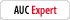# "12:00am" time entry calculation problem

sjsmith
Registered: Sep 2 2008
Posts: 28

I've posted before about a Time Sheet that I am creating. Users enter a date, a Time In and a Time Out. Javascript does calculations as to how many hours they work (rounded off to the quarter hour).

My problem is when users enter "12:00am" (or any minutes with 12:__am). Javascript is reading that as 12:00am the same day. But, if Users enter 00:00, it works fine.

I tried playing around with setting the date and adding a day onto it if the TimeOut value is smaller than the TimeIn value, but my depth of understanding in Javascript prevented me from totally reworking the calculations for that.

I am currently trying to figure out how to have Javascript replace a users entry of "12:00am" with "00.00" but I am not working it out.

Does anyone have any specific suggestions as to how I can fix this problem?

Here's the script for the text field that generates the total number of hours worked in a day.

// creates date time object for start time
var oStart = util.scand("yyyy/mm/dd hh:mm", util.printd("yyyy/mm/dd ", new Date()) + this.getField("TimeIn4").value);

// creates date time object for end time
var oEnd = util.scand("yyyy/mm/dd hh:mm", util.printd("yyyy/mm/dd ", new Date()) + this.getField("TimeOut4").value);

// computes difference in hours
var DiffHours = (oEnd.valueOf() - oStart.valueOf()) / 1000 / 60 / 60;
if (oEnd.valueOf() < oStart.valueOf()) DiffHours = (DiffHours + 24);
var WholeHours = Math.floor(DiffHours);
var DecHours = util.printf("%,302.0f",((DiffHours % 1) * 4).toFixed()) * 25;
var HourTotal = (WholeHours) + "." + (DecHours);
if (DecHours == 100) event.value = Math.ceil(HourTotal);
else event.value = (HourTotal)

Thanks in advance for any assistance.

My Product Information:
Acrobat Pro 7.0.7, Windows
thompRegistered: Feb 15 2006
Posts: 4411
Seems to me that ideally you need to have the user enter a date.

But, otherwise I think your proposed solution is perfect, i.e., add a day if the start time is less than the end time, is perfect.

This part of your calculation if fine
`var DiffHours = (oEnd.valueOf() - oStart.valueOf()) / 1000 / 60 / 60;if (oEnd.valueOf() < oStart.valueOf())DiffHours = (DiffHours + 24);`

to round to a quarter hour try this code.

`DecHours = Math.round((DiffHours - Math.floor(DiffHours))/.25)*.25;DiffHours = Math.floor(DiffHours) + DecHours;`

You can also simplify your time scan code. Since the code is doing a difference, it's only necessary to start from a specific date, The actual date is unimportant.

`var oEnd = util.scand("yy/mm/dd hh:MM", "70/01/01 " + this.getField("TimeOut4").value);`
Thom Parker
The source for PDF Scripting Info
[url=http://www.pdfScripting.com]pdfscripting.com[/url]

The Acrobat JavaScript Reference, Use it Early and Often